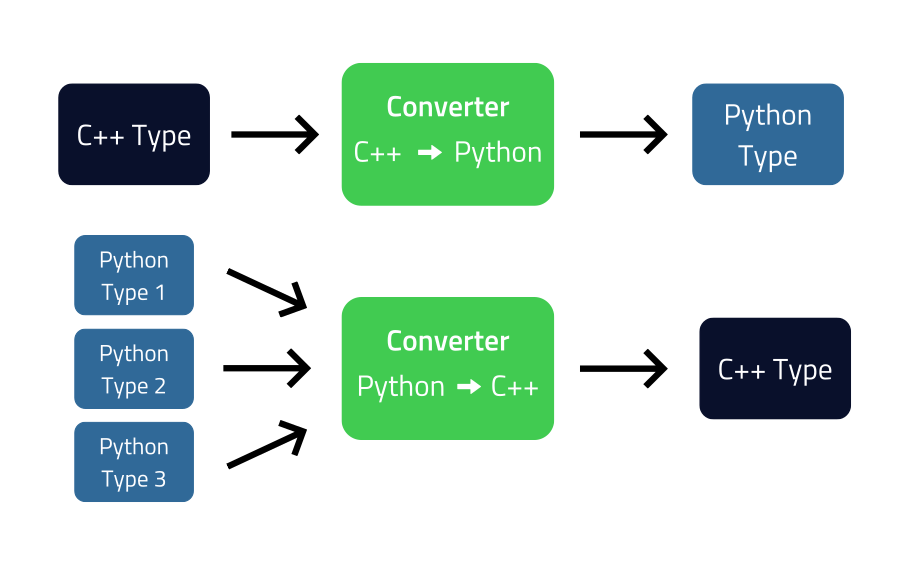User Defined Type Conversion¶

In the process of creating Python bindings of a C++ library, most of the C++ classes will have wrappers representing them in Python land. But there may be other classes that are very simple and/or have a Python type as a direct counter part. (Example: a “Complex” class, that represents complex numbers, has a Python equivalent in the “complex” type.) Such classes, instead of getting a Python wrapper, normally have conversions rules, from Python to C++ and vice-versa.

// C++ class
struct Complex {
Complex(double real, double imag);
double real() const;
double imag() const;
};

// Converting from C++ to Python using the CPython API:
PyObject* pyCpxObj = PyComplex_FromDoubles(complex.real(), complex.imag());

// Converting from Python to C++:
double real = PyComplex_RealAsDouble(pyCpxObj);
double imag = PyComplex_ImagAsDouble(pyCpxObj);
Complex cpx(real, imag);

For the user defined conversion code to be inserted in the proper places, the conversion-rule tag must be used.

<primitive-type name="Complex" target-lang-api-name="PyComplex">
<include file-name="complex.h" location="global"/>

<conversion-rule>

<native-to-target>
return PyComplex_FromDoubles(%in.real(), %in.imag());
</native-to-target>

<target-to-native>
<!-- The 'check' attribute can be derived from the 'type' attribute,
it is defined here to test the CHECKTYPE type system variable. -->
double real = PyComplex_RealAsDouble(%in);
double imag = PyComplex_ImagAsDouble(%in);
%out = %OUTTYPE(real, imag);
</target-to-native>

</conversion-rule>

</primitive-type>

The details will be given later, but the gist of it are the tags native-to-target, which has only one conversion from C++ to Python, and native-to-native, that may define the conversion of multiple Python types to C++’s “Complex” type.Shiboken expects the code for native-to-target, to directly return the Python result of the conversion, and the added conversions inside the target-to-native must attribute the Python to C++ conversion result to the %out variable.

Expanding on the last example, if the binding developer want a Python 2-tuple of numbers to be accepted by wrapped C++ functions with “Complex” arguments, an add-conversion tag and a custom check must be added. Here’s how to do it:

<!-- Code injection at module level. -->
<inject-code class="native" position="beginning">
static bool Check2TupleOfNumbers(PyObject* pyIn) {
if (!PySequence_Check(pyIn) || !(PySequence_Size(pyIn) == 2))
return false;
Shiboken::AutoDecRef pyReal(PySequence_GetItem(pyIn, 0));
if (!PyNumber_Check(pyReal))
return false;
Shiboken::AutoDecRef pyImag(PySequence_GetItem(pyIn, 1));
if (!PyNumber_Check(pyImag))
return false;
return true;
}
</inject-code>

<primitive-type name="Complex" target-lang-api-name="PyComplex">
<include file-name="complex.h" location="global"/>

<conversion-rule>

<native-to-target>
return PyComplex_FromDoubles(%in.real(), %in.imag());
</native-to-target>

<target-to-native>

double real = PyComplex_RealAsDouble(%in);
double imag = PyComplex_ImagAsDouble(%in);
%out = %OUTTYPE(real, imag);

Shiboken::AutoDecRef pyReal(PySequence_GetItem(%in, 0));
Shiboken::AutoDecRef pyImag(PySequence_GetItem(%in, 1));
double real = %CONVERTTOCPP[double](pyReal);
double imag  = %CONVERTTOCPP[double](pyImag);
%out = %OUTTYPE(real, imag);

</target-to-native>

</conversion-rule>

</primitive-type>

Container Conversions¶

Converters for container-type are pretty much the same as for other type, except that they make use of the type system variables %INTYPE_# and %OUTTYPE_#. Shiboken combines the conversion code for containers with the conversion defined (or automatically generated) for the containers.

<container-type name="std::map" type="map">
<include file-name="map" location="global"/>

<conversion-rule>

<native-to-target>
PyObject* %out = PyDict_New();
%INTYPE::const_iterator it = %in.begin();
for (; it != %in.end(); ++it) {
%INTYPE_0 key = it->first;
%INTYPE_1 value = it->second;
PyDict_SetItem(%out,
%CONVERTTOPYTHON[%INTYPE_0](key),
%CONVERTTOPYTHON[%INTYPE_1](value));
}
return %out;
</native-to-target>

<target-to-native>

PyObject* key;
PyObject* value;
Py_ssize_t pos = 0;
while (PyDict_Next(%in, &amp;pos, &amp;key, &amp;value)) {
%OUTTYPE_0 cppKey = %CONVERTTOCPP[%OUTTYPE_0](key);
%OUTTYPE_1 cppValue = %CONVERTTOCPP[%OUTTYPE_1](value);
%out.insert(%OUTTYPE::value_type(cppKey, cppValue));
}

</target-to-native>
</conversion-rule>
</container-type>

Variables & Functions¶

%in

Variable replaced by the C++ input variable.

%out

Variable replaced by the C++ output variable. Needed to convey the result of a Python to C++ conversion.

%INTYPE

Used in Python to C++ conversions. It is replaced by the name of type for which the conversion is being defined. Don’t use the type’s name directly.

%INTYPE_#

Replaced by the name of the #th type used in a container.

%OUTTYPE

Used in Python to C++ conversions. It is replaced by the name of type for which the conversion is being defined. Don’t use the type’s name directly.

%OUTTYPE_#

Replaced by the name of the #th type used in a container.

%CHECKTYPE[CPPTYPE]

Replaced by a Shiboken type checking function for a Python variable. The C++ type is indicated by CPPTYPE.

Converting The Old Converters¶

If you use Shiboken for your bindings, and has defined some type conversions using the Shiboken::Converter template, then you must update your converters to the new scheme.

Previously your conversion rules were declared in one line, like this:

<primitive-type name="Complex" target-lang-api-name="PyComplex">
<include file-name="complex.h" location="global"/>
<conversion-rule file="complex_conversions.h"/>
</primitive-type>

And implemented in a separate C++ file, like this:

namespace Shiboken {
template<> struct Converter<Complex>
{
static inline bool checkType(PyObject* pyObj) {
return PyComplex_Check(pyObj);
}
static inline bool isConvertible(PyObject* pyObj) {
return PyComplex_Check(pyObj);
}
static inline PyObject* toPython(void* cppobj) {